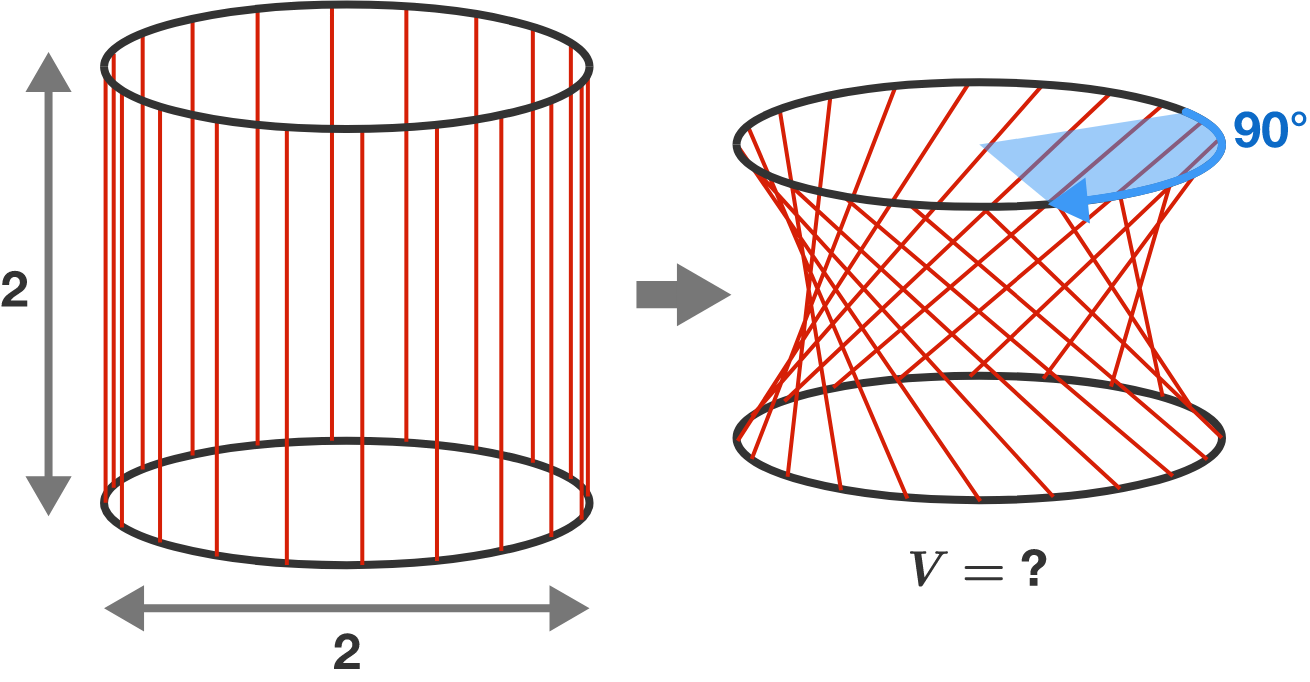# Rotated strings volume

Calculus Level 2

Two unit circles are joined by many equally spaced strings of length $2$ to form a cylinder.

The upper circle is rotated by $90$ degrees while the lower is fixed. If there were infinitely many strings, they would serve as the surface of a 3D figure, together with the two circles.

What is the volume inside this 3D figure?×